# twoRayChannel

Two-ray propagation channel

Since R2021a

## Description

The twoRayChannel models a narrowband two-ray propagation channel. A two-ray propagation channel is the simplest type of multipath channel. You can use a two-ray channel to simulate propagation of signals in a homogeneous, isotropic medium with a single reflecting boundary. This type of medium has two propagation paths: a line-of-sight (direct) propagation path from one point to another and a ray path reflected from the boundary. You can use this System object™ for short-range radar and mobile communications applications where the signals propagate along straight paths and the earth is assumed to be flat. You can also use this object for sonar and microphone applications. For acoustic applications, you can choose the fields to be non-polarized and adjust the propagation speed to be the speed of sound in air or water. You can use twoRayChannel to model propagation from several points simultaneously.

While the System object works for all frequencies, the attenuation models for atmospheric gases and rain are valid for electromagnetic signals in the frequency range 1–1000 GHz only. The attenuation model for fog and clouds is valid for 10–1000 GHz. Outside these frequency ranges, the System object uses the nearest valid value.

The twoRayChannel System object applies range-dependent time delays to the signals, and as well as gains or losses, phase shifts, and boundary reflection loss. The System object applies Doppler shift when either the source or destination is moving.

Signals at the channel output can be kept separate or be combined — controlled by the CombinedRaysOutput property. In the separate option, both fields arrive at the destination separately and are not combined. For the combined option, the two signals at the source propagate separately but are coherently summed at the destination into a single quantity. This option is convenient when the difference between the sensor or array gains in the directions of the two paths is not significant and need not be taken into account.

Unlike the phased.FreeSpace System object, the twoRayChannel System object does not support two-way propagation.

To perform two-ray channel propagation:

1. Create the twoRayChannel object and set its properties.

2. Call the object with arguments, as if it were a function.

## Creation

### Syntax

channel = twoRayChannel
channel = twoRayChannel(Name,Value)

### Description

channel = twoRayChannel creates a two-ray propagation channel System object.

example

channel = twoRayChannel(Name,Value) creates a two-ray propagation channel System object with each specified property Name set to the specified Value. You can specify additional name and value pair arguments in any order as (Name1,Value1,...,NameN,ValueN).

## Properties

expand all

Unless otherwise indicated, properties are nontunable, which means you cannot change their values after calling the object. Objects lock when you call them, and the release function unlocks them.

If a property is tunable, you can change its value at any time.

Signal propagation speed, specified as a positive scalar. Units are in meters per second. The default propagation speed is the value returned by physconst('LightSpeed'). See physconst for more information.

Example: 3e8

Data Types: double

Operating frequency, specified as a positive scalar. Units are in Hz.

Example: 1e9

Data Types: double

Option to enable the atmospheric attenuation model, specified as a false or true. Set this property to true to add signal attenuation caused by atmospheric gases, rain, fog, or clouds. Set this property to false to ignore atmospheric effects in propagation.

Setting SpecifyAtmosphere to true, enables the Temperature, DryAirPressure, WaterVapourDensity, LiquidWaterDensity, and RainRate properties.

Data Types: logical

Ambient temperature, specified as a real-valued scalar. Units are in degrees Celsius.

Example: 20.0

#### Dependencies

To enable this property, set SpecifyAtmosphere to true.

Data Types: double

Atmospheric dry air pressure, specified as a positive real-valued scalar. Units are in pascals (Pa). The default value of this property corresponds to one standard atmosphere.

Example: 101.0e3

#### Dependencies

To enable this property, set SpecifyAtmosphere to true.

Data Types: double

Atmospheric water vapor density, specified as a positive real-valued scalar. Units are in g/m3.

Example: 7.4

#### Dependencies

To enable this property, set SpecifyAtmosphere to true.

Data Types: double

Liquid water density of fog or clouds, specified as a nonnegative real-valued scalar. Units are in g/m3. Typical values for liquid water density are 0.05 for medium fog and 0.5 for thick fog.

Example: 0.1

#### Dependencies

To enable this property, set SpecifyAtmosphere to true.

Data Types: double

Rainfall rate, specified as a nonnegative scalar. Units are in mm/hr.

Example: 10.0

#### Dependencies

To enable this property, set SpecifyAtmosphere to true.

Data Types: double

Sample rate of signal, specified as a positive scalar. Units are in Hz. The System object uses this quantity to calculate the propagation delay in units of samples.

Example: 1e6

Data Types: double

Option to enable polarized fields, specified as false or true. Set this property to true to enable polarization. Set this property to false to ignore polarization.

Data Types: logical

Ground reflection coefficient for the field at the reflection point, specified as a complex-valued scalar or a complex-valued 1-by-N row vector. Each coefficient has an absolute value less than or equal to one. The quantity N is the number of two-ray channels. Units are dimensionless. Use this property to model nonpolarized signals. To model polarized signals, use the GroundRelativePermittivity property.

Example: -0.5

#### Dependencies

To enable this property, set EnablePolarization to false.

Data Types: double
Complex Number Support: Yes

Relative permittivity of the ground at the reflection point, specified as a positive real-valued scalar or a 1-by-N real-valued row vector of positive values. The dimension N is the number of two-ray channels. Permittivity units are dimensionless. Relative permittivity is defined as the ratio of actual ground permittivity to the permittivity of free space. This property applies when you set the EnablePolarization property to true. Use this property to model polarized signals. To model nonpolarized signals, use the GroundReflectionCoefficient property.

Example: 5

#### Dependencies

To enable this property, set EnablePolarization to true.

Data Types: double

Option to combine the two rays at channel output, specified as true or false. When this property is true, the object coherently adds the line-of-sight propagated signal and the reflected path signal when forming the output signal. Use this mode when you do not need to include the directional gain of an antenna or array in your simulation.

Data Types: logical

Source of maximum one-way propagation distance, specified as 'Auto' or 'Property'. The maximum one-way propagation distance is used to allocate sufficient memory for signal delay computation. When you set this property to 'Auto', the System object automatically allocates memory. When you set this property to 'Property', you specify the maximum one-way propagation distance using the value of the MaximumDistance property.

Data Types: char

Maximum one-way propagation distance, specified as a positive real-valued scalar. Units are in meters. Any signal that propagates more than the maximum one-way distance is ignored. The maximum distance must be greater than or equal to the largest position-to-position distance.

Example: 5000

#### Dependencies

To enable this property, set the MaximumDistanceSource property to 'Property'.

Data Types: double

The source of the maximum number of samples of the input signal, specified as 'Auto' or 'Property'. When you set this property to 'Auto', the propagation model automatically allocates enough memory to buffer the input signal. When you set this property to 'Property', you specify the maximum number of samples in the input signal using the MaximumNumInputSamples property. Any input signal longer than that value is truncated.

To use this object with variable-size signals in a MATLAB® Function Block in Simulink®, set the MaximumNumInputSamplesSource property to 'Property' and set a value for the MaximumNumInputSamples property.

Example: 'Property'

#### Dependencies

To enable this property, set MaximumDistanceSource to 'Property'.

Data Types: char

Maximum number of input signal samples, specified as a positive integer. The size of the input signal is the number of rows in the input matrix. Any input signal longer than this number is truncated. To process signals completely, ensure that this property value is greater than any maximum input signal length.

The waveform-generating System objects determine the maximum signal size:

• For any waveform, if the waveform OutputFormat property is set to 'Samples', the maximum signal length is the value specified in the NumSamples property.

• For pulse waveforms, if the OutputFormat is set to 'Pulses', the signal length is the product of the smallest pulse repetition frequency, the number of pulses, and the sample rate.

• For continuous waveforms, if the OutputFormat is set to 'Sweeps', the signal length is the product of the sweep time, the number of sweeps, and the sample rate.

Example: 2048

#### Dependencies

To enable this property, set MaximumNumInputSamplesSource to 'Property'.

Data Types: double

## Usage

### Syntax

prop_sig = channel(sig,origin_pos,dest_pos,origin_vel,dest_vel)

### Description

example

prop_sig = channel(sig,origin_pos,dest_pos,origin_vel,dest_vel) returns the resulting signal, prop_sig, when a narrowband signal, sig, propagates through a two-ray channel from the origin_pos position to the dest_pos position. Either the origin_pos or dest_pos arguments can have multiple points but you cannot specify both as having multiple points. The velocity of the signal origin is specified in origin_vel and the velocity of the signal destination is specified in dest_vel. The dimensions of origin_vel and dest_vel must agree with the dimensions of origin_pos and dest_pos, respectively.Electromagnetic fields propagated through a two-ray channel can be polarized or nonpolarized. For, nonpolarized fields, such as an acoustic field, the propagating signal field, sig, is a vector or matrix. When the fields are polarized, sig is an array of structures. Every structure element represents an electric field vector in Cartesian form.In the two-ray environment, there are two signal paths connecting every signal origin and destination pair. For N signal origins (or N signal destinations), there are 2N number of paths. The signals for each origin-destination pair do not have to be related. The signals along the two paths for any single source-destination pair can also differ due to phase or amplitude differences.You can keep the two signals at the destination separate or combined — controlled by the CombinedRaysOutput property. Combined means that the signals at the source propagate separately along the two paths but are coherently summed at the destination into a single quantity. To use the separate option, set CombinedRaysOutput to false. To use the combined option, set CombinedRaysOutput to true. This option is convenient when the difference between the sensor or array gains in the directions of the two paths is not significant and need not be taken into account.

### Input Arguments

expand all

• Narrowband nonpolarized scalar signal, specified as an

• M-by-N complex-valued matrix. Each column contains a common signal propagated along both the line-of-sight path and the reflected path. You can use this form when both path signals are the same.

• M-by-2N complex-valued matrix. Each adjacent pair of columns represents a different channel. Within each pair, the first column represents the signal propagated along the line-of-sight path and the second column represents the signal propagated along the reflected path.

• Narrowband polarized signal, specified as a

• 1-by-N struct array containing complex-valued fields. Each struct contains a common polarized signal propagated along both the line-of-sight path and the reflected path. Each structure element contains an M-by-1 column vector of electromagnetic field components (sig.X,sig.Y,sig.Z). You can use this form when both path signals are the same.

• 1-by-2N struct array containing complex-valued fields. Each adjacent pair of array columns represents a different channel. Within each pair, the first column represents the signal along the line-of-sight path and the second column represents the signal along the reflected path. Each structure element contains an M-by-1 column vector of electromagnetic field components (sig.X,sig.Y,sig.Z).

For nonpolarized fields, the quantity M is the number of samples of the signal and N is the number of two-ray channels. Each channel corresponds to a source-destination pair.

The size of the first dimension of the input matrix can vary to simulate a changing signal length. A size change can occur, for example, in the case of a pulse waveform with variable pulse repetition frequency.

For polarized fields, the struct element contains three M-by-1 complex-valued column vectors, sig.X, sig.Y, and sig.Z. These vectors represent the x, y, and z Cartesian components of the polarized signal.

The size of the first dimension of the matrix fields within the struct can vary to simulate a changing signal length such as a pulse waveform with variable pulse repetition frequency.

Example: [1,1;j,1;0.5,0]

Data Types: double
Complex Number Support: Yes

Origin of the signal or signals, specified as a 3-by-1 real-valued column vector or 3-by-N real-valued matrix. The quantity N is the number of two-ray channels. If origin_pos is a column vector, it takes the form [x;y;z]. If origin_pos is a matrix, each column specifies a different signal origin and has the form [x;y;z]. Position units are meters.

origin_pos and dest_pos cannot both be specified as matrices — at least one must be a 3-by-1 column vector.

Example: [1000;100;500]

Data Types: double

Destination position of the signal or signals, specified as a 3-by-1 real-valued column vector or 3-by-N real-valued matrix. The quantity N is the number of two-ray channels propagating from or to N signal origins. If dest_pos is a 3-by-1 column vector, it takes the form [x;y;z]. If dest_pos is a matrix, each column specifies a different signal destination and takes the form [x;y;z] Position units are in meters.

You cannot specify origin_pos and dest_pos as matrices. At least one must be a 3-by-1 column vector.

Example: [0;0;0]

Data Types: double

Velocity of signal origin, specified as a 3-by-1 real-valued column vector or 3-by-N real-valued matrix. The dimensions of origin_vel must match the dimensions of origin_pos. If origin_vel is a column vector, it takes the form [Vx;Vy;Vz]. If origin_vel is a 3-by-N matrix, each column specifies a different origin velocity and has the form [Vx;Vy;Vz]. Velocity units are in meters per second.

Example: [10;0;5]

Data Types: double

Velocity of signal destinations, specified as a 3-by-1 real-valued column vector or 3–by-N real-valued matrix. The dimensions of dest_vel must match the dimensions of dest_pos. If dest_vel is a column vector, it takes the form [Vx;Vy;Vz]. If dest_vel is a 3-by-N matrix, each column specifies a different destination velocity and has the form [Vx;Vy;Vz] Velocity units are in meters per second.

Example: [0;0;0]

Data Types: double

### Output Arguments

expand all

• Narrowband nonpolarized scalar signal, returned as an:

• M-by-N complex-valued matrix. To return this format, set the CombinedRaysOutput property to true. Each matrix column contains the coherently combined signals from the line-of-sight path and the reflected path.

• M-by-2N complex-valued matrix. To return this format set the CombinedRaysOutput property to false. Alternate columns of the matrix contain the signals from the line-of-sight path and the reflected path.

• Narrowband polarized scalar signal, returned as:

• 1-by-N struct array containing complex-valued fields. To return this format, set the CombinedRaysOutput property to true. Each column of the array contains the coherently combined signals from the line-of-sight path and the reflected path. Each structure element contains the electromagnetic field vector (prop_sig.X,prop_sig.Y,prop_sig.Z).

• 1-by-2N struct array containing complex-valued fields. To return this format, set the CombinedRaysOutput property to false. Alternate columns contains the signals from the line-of-sight path and the reflected path. Each structure element contains the electromagnetic field vector (prop_sig.X,prop_sig.Y,prop_sig.Z).

The output prop_sig contains signal samples arriving at the signal destination within the current input time frame. Whenever it takes longer than the current time frame for the signal to propagate from the origin to the destination, the output may not contain all contributions from the input of the current time frame. The remaining output will appear in the next call to the object.

## Object Functions

To use an object function, specify the System object as the first input argument. For example, to release system resources of a System object named obj, use this syntax:

release(obj)

expand all

 step Run System object algorithm release Release resources and allow changes to System object property values and input characteristics reset Reset internal states of System object

## Examples

collapse all

This example illustrates the two-ray propagation of a signal, showing how the signals from the line-of-sight and reflected path arrive at the receiver at different times.

Create and Plot Propagating Signal

Create a nonpolarized electromagnetic field consisting of two rectangular waveform pulses at a carrier frequency of 100 MHz. Assume the pulse width is 10 ms and the sampling rate is 1 MHz. The bandwidth of the pulse is 0.1 MHz. Assume a 50% duty cycle in so that the pulse width is one-half the pulse repetition interval. Create a two-pulse wave train. Set the GroundReflectionCoefficient to 0.9 to model strong ground reflectivity. Propagate the field from a stationary source to a stationary receiver. The vertical separation of the source and receiver is approximately 10 km.

c = physconst('LightSpeed'); fs = 1e6; pw = 10e-6; pri = 2*pw; PRF = 1/pri; fc = 100e6; lambda = c/fc; waveform = phased.RectangularWaveform('SampleRate',fs,'PulseWidth',pw,... 'PRF',PRF,'OutputFormat','Pulses','NumPulses',2); wav = waveform(); n = size(wav,1); figure; plot((0:(n-1)),real(wav),'b.-'); xlabel('Time (samples)') ylabel('Waveform magnitude')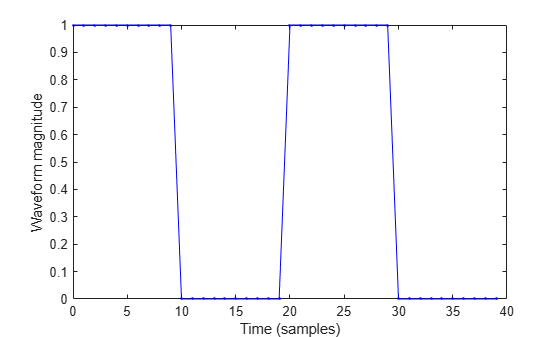Specify the Location of Source and Receiver

Place the source and receiver about 1000 meters apart horizontally and approximately 10 km apart vertically.

pos1 = [1000;0;10000]; pos2 = [0;100;100]; vel1 = [0;0;0]; vel2 = [0;0;0];

Compute the predicted signal delays in units of samples.

[rng,ang] = rangeangle(pos2,pos1,'two-ray');

Create a Two-Ray Channel System Object™

Create a two-ray propagation channel System object™ and propagate the signal along both the line-of-sight and reflected ray paths.

channel = twoRayChannel('SampleRate',fs,... 'GroundReflectionCoefficient',.9,'OperatingFrequency',fc,... 'CombinedRaysOutput',false); prop_signal = channel([wav,wav],pos1,pos2,vel1,vel2);

Plot the Propagated Signals

• Plot the signal propagated along the line-of-sight.

• Then, overlay a plot of the signal propagated along the reflected path.

• Finally, overlay a plot of the coherent sum of the two signals.

n = size(prop_signal,1); delay = 0:(n-1); plot(delay,abs(prop_signal(:,1)),'g') hold on plot(delay,abs(prop_signal(:,2)),'r') plot(delay,abs(prop_signal(:,1) + prop_signal(:,2)),'b') hold off legend('Line-of-sight','Reflected','Combined','Location','NorthWest') xlabel('Delay (samples)') ylabel('Signal Magnitude')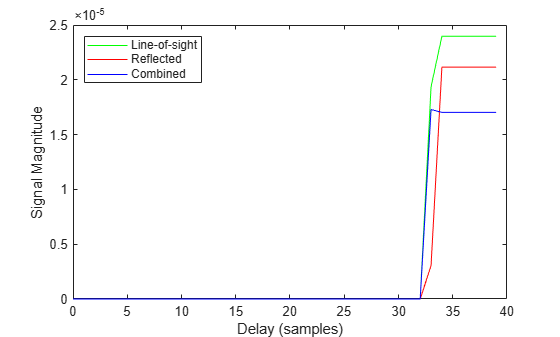The plot shows that the delay of the reflected path signal agrees with the predicted delay. The magnitude of the coherently combined signal is less than either of the propagated signals indicating that there is some interference between the two signals.

Create a polarized electromagnetic field consisting of linear FM waveform pulses. Propagate the field from a stationary source with a crossed-dipole antenna element to a stationary receiver approximately 10 km away. The transmitting antenna is 100 meters above the ground. The receiving antenna is 150 m above the ground. The receiving antenna is also a crossed-dipole. Plot the received signal.

Assume the pulse width is $10\mu s$ and the sampling rate is 10 MHz. The bandwidth of the pulse is 1 MHz. Assume a 50% duty cycle in which the pulse width is one-half the pulse repetition interval. Create a two-pulse wave train. Assume a carrier frequency of 100 MHz.

c = physconst('LightSpeed'); fs = 10e6; pw = 10e-6; pri = 2*pw; PRF = 1/pri; fc = 100e6; bw = 1e6; lambda = c/fc;

Set Up Required System Objects

Use a GroundRelativePermittivity of 10.

waveform = phased.LinearFMWaveform('SampleRate',fs,'PulseWidth',pw,... 'PRF',PRF,'OutputFormat','Pulses','NumPulses',2,'SweepBandwidth',bw,... 'SweepDirection','Up','Envelope','Rectangular','SweepInterval',... 'Positive'); antenna = phased.CrossedDipoleAntennaElement(... 'FrequencyRange',[50,200]*1e6); radiator = phased.Radiator('Sensor',antenna,'OperatingFrequency',fc,... 'Polarization','Combined'); channel = twoRayChannel('SampleRate',fs,... 'OperatingFrequency',fc,'CombinedRaysOutput',false,... 'EnablePolarization',true,'GroundRelativePermittivity',10); collector = phased.Collector('Sensor',antenna,'OperatingFrequency',fc,... 'Polarization','Combined');

Set Up Scene Geometry

Specify transmitter and receiver positions, velocities, and orientations. Place the source and receiver about 1000 m apart horizontally and approximately 50 m apart vertically.

posTx = [0;100;100]; posRx = [1000;0;150]; velTx = [0;0;0]; velRx = [0;0;0]; laxRx = rotz(180); laxTx = rotx(1)*eye(3);

Create and Radiate Signals from Transmitter

Compute the transmission angles for the two rays traveling toward the receiver. These angles are defined with respect to the transmitter local coordinate system. The phased.Radiator System object™ uses these angles to apply separate antenna gains to the two signals.

[rng,angsTx] = rangeangle(posRx,posTx,laxTx,'two-ray'); wav = waveform();

Plot the transmitted Waveform

n = size(wav,1); plot((0:(n-1))/fs*1000000,real(wav)) xlabel('Time ({\mu}sec)') ylabel('Waveform')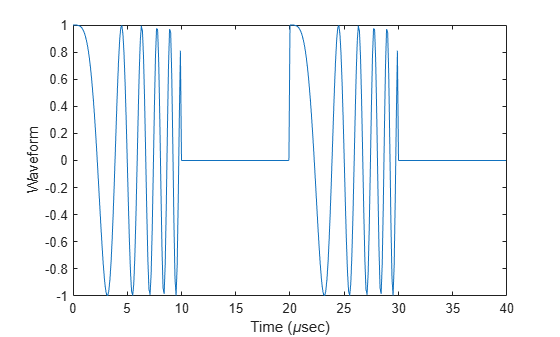sig = radiator(wav,angsTx,laxTx);

Propagate signals to receiver via two-ray channel

prop_sig = channel(sig,posTx,posRx,velTx,velRx);

Compute the reception angles for the two rays arriving at the receiver. These angles are defined with respect to the receiver local coordinate system. The phased.Collector System object™ uses these angles to apply separate antenna gains to the two signals.

[~,angsRx] = rangeangle(posTx,posRx,laxRx,'two-ray');

y = collector(prop_sig,angsRx,laxRx);

plot((0:(n-1))/fs*1000000,real(y)) xlabel('Time ({\mu}sec)') ylabel('Received Waveform')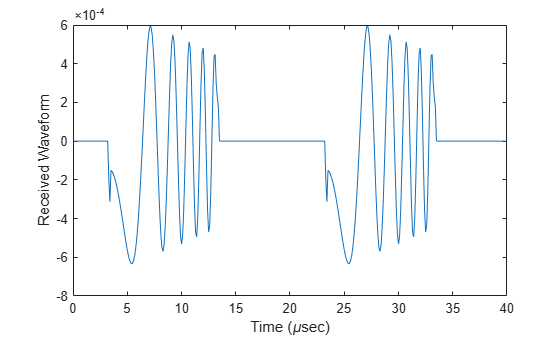Propagate a signal in a two-ray channel environment from a radar at (0,0,10) meters to a target at (300,200,30) meters. Assume that the radar and target are stationary and that the transmitting antenna has a cosine pattern. Compare the combined signals from the two paths with the single signal resulting from free space propagation. Set the CombinedRaysOutput to true to produce a combined propagated signal.

Create a Rectangular Waveform

Set the sample rate to 2 MHz.

fs = 2e6; waveform = phased.RectangularWaveform('SampleRate',fs); wavfrm = waveform();

Create the Transmitting Antenna and Radiator

Set up a phased.Radiator System object™ to transmit from a cosine antenna

antenna = phased.CosineAntennaElement; radiator = phased.Radiator('Sensor',antenna);

Specify Transmitter and Target Coordinates

posTx = [0;0;10]; posTgt = [300;200;30]; velTx = [0;0;0]; velTgt = [0;0;0];

Free Space Propagation

Compute the transmitting direction toward the target for the free-space model. Then, radiate the signal.

[~,angFS] = rangeangle(posTgt,posTx); wavTx = radiator(wavfrm,angFS);

Propagate the signal to the target.

fschannel = phased.FreeSpace('SampleRate',waveform.SampleRate); yfs = fschannel(wavTx,posTx,posTgt,velTx,velTgt); release(radiator);

Two-Ray Propagation

Compute the two transmit angles toward the target for line-of-sight (LOS) path and reflected paths. Compute the transmitting directions toward the target for the two rays. Then, radiate the signals.

[~,angTwoRay] = rangeangle(posTgt,posTx,'two-ray'); wavTwoRay = radiator(wavfrm,angTwoRay);

Propagate the signals to the target.

channel = twoRayChannel('SampleRate',waveform.SampleRate,... 'CombinedRaysOutput',true); y2ray = channel(wavTwoRay,posTx,posTgt,velTx,velTgt);

Plot the Propagated Signals

Plot the combined signal against the free-space signal

plot(abs([y2ray yfs])) legend('Two-ray','Free space') xlabel('Samples') ylabel('Signal Magnitude')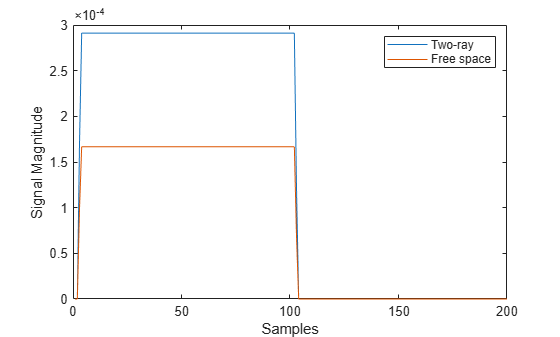Propagate a linear FM signal in a two-ray channel. The signal propagates from a transmitter located at (1000,10,10) meters in the global coordinate system to a receiver at (10000,200,30) meters. Assume that the transmitter and the receiver are stationary and that they both have cosine antenna patterns. Plot the received signal.

Set up the radar scenario. First, create the required System objects.

waveform = phased.LinearFMWaveform('SampleRate',1000000,... 'OutputFormat','Pulses','NumPulses',2); fs = waveform.SampleRate; antenna = phased.CosineAntennaElement; radiator = phased.Radiator('Sensor',antenna); collector = phased.Collector('Sensor',antenna); channel = twoRayChannel('SampleRate',fs,... 'CombinedRaysOutput',false,'GroundReflectionCoefficient',0.95);

Set up the scene geometry. Specify transmitter and receiver positions and velocities. The transmitter and receiver are stationary.

posTx = [1000;10;10]; posRx = [10000;200;30]; velTx = [0;0;0]; velRx = [0;0;0];

Specify the transmitting and receiving radar antenna orientations with respect to the global coordinates. The transmitting antenna points along the +x direction and the receiving antenna points near but not directly in the -x direction.

laxTx = eye(3); laxRx = rotx(5)*rotz(170);

Compute the transmission angles which are the angles that the two rays traveling toward the receiver leave the transmitter. The phased.Radiator System object™ uses these angles to apply separate antenna gains to the two signals. Because the antenna gains depend on path direction, you must transmit and receive the two rays separately.

[~,angTx] = rangeangle(posRx,posTx,laxTx,'two-ray');

Create and radiate signals from transmitter along the transmission directions.

wavfrm = waveform(); wavtrans = radiator(wavfrm,angTx);

Propagate signals to receiver via two-ray channel.

wavrcv = channel(wavtrans,posTx,posRx,velTx,velRx);

Collect signals at the receiver. Compute the angle at which the two rays traveling from the transmitter arrive at the receiver. The phased.Collector System object™ uses these angles to apply separate antenna gains to the two signals.

[~,angRcv] = rangeangle(posTx,posRx,laxRx,'two-ray');

Collect and combine the two received rays.

yR = collector(wavrcv,angRcv);

dt = 1/fs; n = size(yR,1); plot((0:(n-1))*dt*1000000,real(yR)) xlabel('Time ({\mu}sec)') ylabel('Signal Magnitude')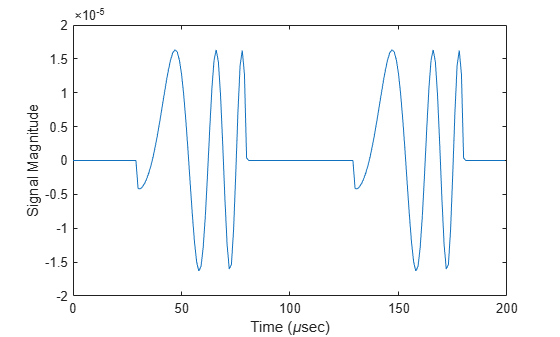Propagate a 100 Mhz linear FM signal into a two-ray channel. Assume there is signal loss caused by atmospheric gases and rain. The signal propagates from a transmitter located at (0,0,0) meters in the global coordinate system to a receiver at (10000,200,30) meters. Assume that the transmitter and the receiver are stationary and that they both have cosine antenna patterns. Plot the received signal. Set the dry air pressure to 102.5 Pa and the rain rate to 5 mm/hr.

waveform = phased.LinearFMWaveform('SampleRate',1e6,... 'OutputFormat','Pulses','NumPulses',2); antenna = phased.CosineAntennaElement; radiator = phased.Radiator('Sensor',antenna); collector = phased.Collector('Sensor',antenna); channel = twoRayChannel('SampleRate',waveform.SampleRate,... 'CombinedRaysOutput',false,'GroundReflectionCoefficient',0.95,... 'SpecifyAtmosphere',true,'Temperature',20,... 'DryAirPressure',102.5,'RainRate',5.0);

Set up the scene geometry giving. the transmitter and receiver positions and velocities. The transmitter and receiver are stationary.

posTx = [0;0;0]; posRx = [10000;200;30]; velTx = [0;0;0]; velRx = [0;0;0];

Specify the transmitting and receiving radar antenna orientations with respect to the global coordinates. The transmitting antenna points along the +x-direction and the receiving antenna points close to the –x-direction.

laxTx = eye(3); laxRx = rotx(5)*rotz(170);

Compute the transmission angles which are the angles that the two rays traveling toward the receiver leave the transmitter. The phased.Radiator System object™ uses these angles to apply separate antenna gains to the two signals. Because the antenna gains depend on path direction, you must transmit and receive the two rays separately.

[~,angTx] = rangeangle(posRx,posTx,laxTx,'two-ray');

Create and Radiate Signals from Transmitter

Radiate the signals along the transmission directions.

wavfrm = waveform(); wavtrans = radiator(wavfrm,angTx);

Propagate signals to receiver via two-ray channel.

wavrcv = channel(wavtrans,posTx,posRx,velTx,velRx);

Compute the angle at which the two rays traveling from the transmitter arrive at the receiver. The phased.Collector System object™ uses these angles to apply separate antenna gains to the two signals.

[~,angRcv] = rangeangle(posTx,posRx,laxRx,'two-ray');

Collect and combine the two received rays.

yR = collector(wavrcv,angRcv);

dt = 1/waveform.SampleRate; n = size(yR,1); plot((0:(n-1))*dt*1000000,real(yR)) xlabel('Time ({\mu}sec)') ylabel('Signal Magnitude')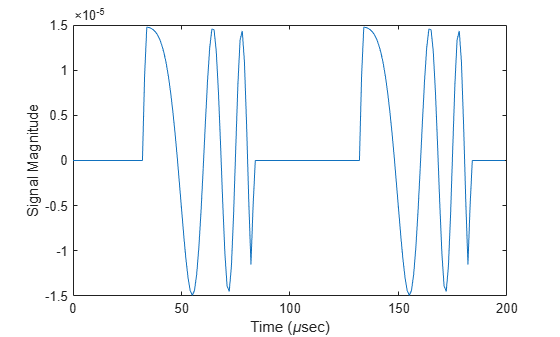expand all

 Saakian, A. Radio Wave Propagation Fundamentals. Norwood, MA: Artech House, 2011.

 Balanis, C. Advanced Engineering Electromagnetics. New York: Wiley & Sons, 1989.

 Rappaport, T. Wireless Communications: Principles and Practice, 2nd Ed New York: Prentice Hall, 2002.

 Radiocommunication Sector of the International Telecommunication Union. Recommendation ITU-R P.676-10: Attenuation by atmospheric gases. 2013.

 Radiocommunication Sector of the International Telecommunication Union. Recommendation ITU-R P.840-6: Attenuation due to clouds and fog. 2013.

 Radiocommunication Sector of the International Telecommunication Union. Recommendation ITU-R P.838-3: Specific attenuation model for rain for use in prediction methods. 2005.